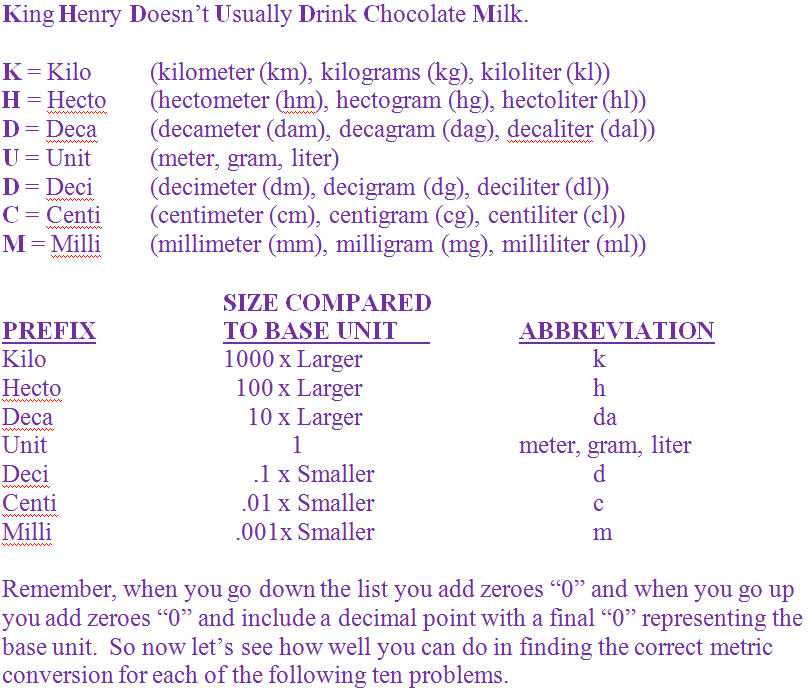Weights are measured in grams.

# Measurement - The Metric System - Easy Conversions 2

This Math quiz is called 'Measurement - The Metric System - Easy Conversions 2' and it has been written by teachers to help you if you are studying the subject at middle school. Playing educational quizzes is a fabulous way to learn if you are in the 6th, 7th or 8th grade - aged 11 to 14.

It costs only \$12.50 per month to play this quiz and over 3,500 others that help you with your school work. You can subscribe on the page at Join Us

This quiz will continue from Easy Conversions [Part 1]. It will help you to become more comfortable with the basic understanding of how the metric system works. Remember, the metric system works in base 10 conversions and the measurements for length/distance, mass/weight and volume are meters, grams and liters.

Go straight to Quiz1.
5,000 mg = ___ g
0.005
0.05
0.5
5
As we are moving up from 5,000 milligrams, it is understood that 5,000 milligrams is the same as 5000.0. Moving up we must move the decimal point over one space to the left for each space we go up the metric list. The gram is three spaces up from the milligram so 5000.0 becomes 5.0. 5,000 milligrams (mg) equals 5 grams (g). Answer (d) is the correct answer
2.
47 dag = ___ hg
470
4.7
.47
.047
As we are moving up from 47 decagrams, it is understood that 47 decagrams is the same as 47.0. Moving up we must move the decimal point over one space to the left for each space we go up the metric list. The hectogram is one space up from the decagram so 47.0 becomes 4.7. 47 decagrams (dag) equals 4.7 hectograms (hg). Answer (b) is the correct answer
3.
70 hl = ___ cl
700
70,000
700,000
7,000,000
As we are moving down the metric list we will be adding zeroes “0”. There are four spaces between a hectoliter and a centiliter so 70 hectoliters (hl) equals 700,000 centiliters (cl). Answer (c) is the correct answer
4.
1,048 dam = ___ mm
10,480,000
1,048,000
104,800
10,480
As we are moving down the metric list we will be adding zeroes “0”. There are four spaces between a decameter and a millimeter so 1,048 decameters (dam) equals 10,480,000 millimeters (mm). Answer (a) is the correct answer
5.
6,986 cm = ___ hm
698.6
69.86
0.6986
0.06986
As we are moving up from 6,986 centimeters, it is understood that 6,986 centimeters is the same as 6986.0. Moving up we must move the decimal point over one space to the left for each space we go up the metric list. The hectometer is four spaces up from the centimeter so 6986.0 becomes 0.6986. 6,986 centimeters (cm) equals 0.6986 hectometers (hm). Answer (c) is the correct answer
6.
543 kg = ___ dg
54,300,000
5,430,000
543.000
54,300
As we are moving down the metric list we will be adding zeroes “0”. There are four spaces between a kilogram and a decigram so 543 kilograms (kg) equals 5,430,000 decigrams (dg). Answer (b) is the correct answer
7.
98 l = ___ kl
980
9.8
0.98
0.098
As we are moving up from 98 liters, it is understood that 98 liters is the same as 98.0. Moving up we must move the decimal point over one space to the left for each space we go up the metric list. The kiloliter is three spaces up from the liter so 98.0 becomes 0.098. 98 liters (l) equals 0.098 kiloliters (kl). Answer (d) is the correct answer
8.
500 l = ___ ml
50,000
500,000
5,000,000
50,000,000
As we are moving down the metric list we will be adding zeroes “0”. There are three spaces between a liter and a milliliter so 500 liters (l) equals 500,000 milliliters (ml). Answer (b) is the correct answer
9.
62 m = ___ hm
0.62
620
6.2
6,200
As we are moving up from 62 meters, it is understood that 62 meters is the same as 62.0. Moving up we must move the decimal point over one space to the left for each space we go up the metric list. The hectometer is two spaces up from the meter so 62.0 becomes 0.62. 62 meters (m) equals 0.62 hectometers (hm). Answer (a) is the correct answer
10.
86 g = ___ cg
8.6
860
8,600
86,000
As we are moving down the metric list we will be adding zeroes “0”. There are two spaces between a gram and a centigram so 86 grams (g) equals 8,600 centigrams (cg). Answer (c) is the correct answer
Author:  Christine G. Broome